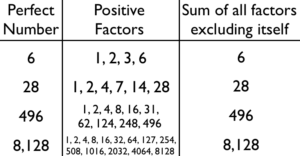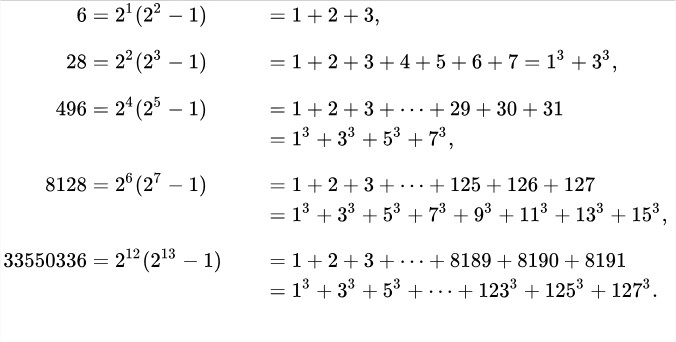# Perfect Number In Java Program – 3 Ways | Programs

Java program to check a perfect number or not. The following program has been written in 3 different ways to check whether the given number is a perfect number or not. The compiler is also added so that you can execute the program yourself, along with sample outputs citing few examples. The methods used are:

• Print using While Loop.
• Print using Static Method.
• Print using Recursion.

A Perfect number is a positive integer that is equal to the sum of its proper divisors except itself.Let’s take an easy example, such as 6.

1, 2, 3 and 6 are the divisors of 6. If we add up all the numbers except 6, we end with the sum of 6 itself.

1 + 2 + 3 = 6 = Perfect Number.

Here are a few more examples of perfect numbers:As you can see, these are regarded as even perfect numbers. An even perfect number is a perfect number that is even, i.e., an even number n whose sum of divisors (including n itself) equals n.

All known perfect numbers are even. Ochem and Rao (2012) have demonstrated that any odd perfect number must be larger than 10^1500.

## Perfect Number – Using While Loop

1) The number which is equal to the sum of its divisors is called a perfect number.

2) Read the entered long number, assigned to the long variable n.

3) while loop iterates until the condition (i<=n/2) is false. If the remainder of n/i=0 then add i value to sum and increase the i value. After all the iterations compare the number with the sum, if both are equal then prints the number is a perfect number.

4) Example n=6,i=1 condition at while is 1<=3 is true, here 6%i=0 is true so sum=1,

i=2, 2<3 is true, 6%2=0 is true so sum=1+2=3,

i=3, 3<=3 is true ,6%3=0 is true so sum=3+3=6, for i=4 the while loop terminate and compare the sum value with n, both are equal, so it prints 6 is a perfect number.

Output:

### Using Static Method

1) In this program we have a static method long perfectOrNot(long num), it calculates the sum of proper divisors of the given number.

2) Call the method long perfectOrNot(long num) in the main method as perfectOrNot(n). Then that method executes the code and returns the sum value, the sum will be assigned to the variable p. Now compare the p with the given number n, if both are equal then it prints a number is a perfect number.

Output:

Perfect Number – Using Recursion

1) Call the method  perfectOrNot() using the Perfect class object as p.perfectOrNot(n,i), then perfectOrNot(n,i) method starts the execution and calls itself as perfectOrNot(num,i); it repeats until the condition i<=num/2 is false.

2) This method returns the sum value and compares the sum with the original number, if both are equal then that number is called a perfect number.

Output:

More Java Programs:

x

## Plus Star Pattern Java Program | Patterns

Java program to print plus star pattern program – We have written the below print/draw ...